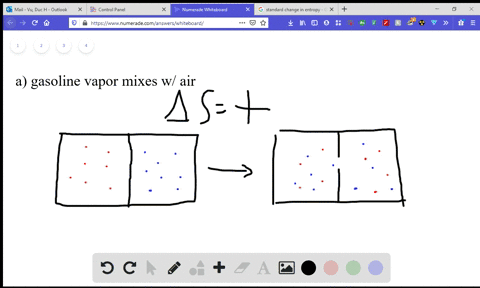Enroll in one of our FREE online STEM summer camps. Space is limited so join now!View Summer Courses### Predict the sign of $\Delta S_{\mathrm{sys}}$ for…

05:02North Carolina State University

Need more help? Fill out this quick form to get professional live tutoring.

Get live tutoring
Problem 12

Predict the sign of $\Delta S_{\mathrm{sys} \text { for each process: (a) A piece of }}$ wax melts. (b) Silver chloride precipitates from solution. (c) Dew forms on a lawn in the morning.

Check back soon!

## Discussion

You must be signed in to discuss.

## Video Transcript

check the 20 Problem 12 is asking us to predict the sign of a change in entropy for various processes. So first, let's define entropy. Injury is the freedom of particle motion or the dispersion of energy. So when we think about these things in the relationships between the two, there greater the entropy, the greater the freedom of particle motion or the greater the dispersion of energy. Let's think about when these things are increasing. When you have an increase in entropy, when you have an increase in interest shown by this air going up for E Or actually let's use this symbol of entropy, which is s when you have an increase in the change of entropy. This happens when you move from a solid from the Solace Day, such as ice to the liquid state, and we're gonna put ella for liquid and then to the gas they. As you move through these phases, the particles have more freedom of motion. They're able to move around more and their energy is further dispersed off. Brought the particles and all throughout the system, you can also have an increase in tribute when you have an increase in temperature so mosque going to use the arrow up for increase, and we have an increase in temperature as well as change from a solid to a liquid to gas. We could have an increase in intra Pete or the greater the freedom of product emotion and greater dispersion and of energy. So let's look at some examples. If we have a piece of wax that is a solid when it melts, it becomes a liquid. Going from a solid state to the liquid state means you're gonna have an increase in entropy. That means the entropy in the beginning. So let's say that an initial entropy is smaller, then the final entropy. And therefore, if our initial entropy is smaller than our final inter Pete, we would have a positive sign. So going from a solid wax to liquid wax is an increase in entropy, and therefore we expect the sign to be positive because we take our final, which is greater, and subtract our initial final minus your initial would give you a greater final might, as a small initial, give you a positive sign. Let's also look at silver chloride, precipitating from solution. That means the silver chloride started off in the liquid form. They began in the liquid form, and then it precipitates out of that solution and becomes a solid. In this case, we go from a liquid to a solid, so we're having a decrease in entropy. That means our initial entropy, which was the liquid state, was greater than our final entropy, which is the solid state. So we'll do the smaller, solid miners, the bit minus the bigger entropy value for our liquid, and we would end up with a negative value. Lastly, let's think about do forming on the lot in the morning. So do comes from water vapour in the air. So when that starts off is a vapour and gas face, and then it condenses to form a liquid phase onto the ground or onto leaves. So we're going from a larger state of Inter Pete to a smaller state of entropy that again means that are larger state and should be. The initial is greater than our final liquid state. So we're going to again end up with a negative entropy. And we're doing this because our the way we're calculating the change of entropy since it's a state function is we're doing the final minus the initial. So if interviews the freedom of particle motion and the dispersion of interest of energy And as you go from a solid to a liquid to a gas that entropy increases if we're thinking about a piece of wax melting that goes from the solid state to the liquid state. So therefore our final minus our initial gets a positive value. If we're going and thinking about silver chloride precipitating from solution, we're going from solution. The initial phase here minus the final phase, which is a solid initial phase. Our final phase. Excuse me. It's smaller than our initial face that will give us a negative sign for our entropy and then similar thing. What happen if we're looking at the dew forming on the law in the morning? Going from the gas phase, which is in greater in tribute to the liquid phase, which is a smaller entropy, smaller value minus a larger value shown here will give you a negative sign